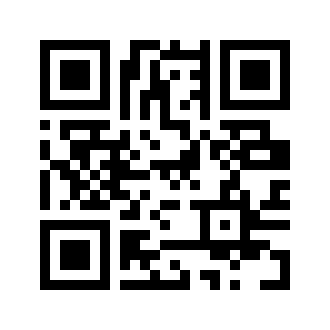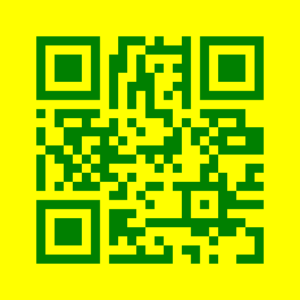# Python qrcode Module with Examples

We can generate our own QR codes using python

QR (Quick Response) code:

QR codes stores a large amount of data and, when scanned, provide fast access to the information.

It saves all data as a series of pixels in a square grid. In general, QR codes are used for the following purposes:

• Making payments

The three big squares outside the QR code are the major components of a conventional QR code. When the QR Reader recognizes them, it knows the entire information stored within the square.

## qrcode Module with Examples in Python

### Using Built-in Functions (Static Input)

Approach:

• Import qrcode module using the import keyword.
• Create an object to the qrcode using the QRCode() function and store it in a variable.
• Add data to the above QRcode using the add_data() function by passing some random string as an argument.
• Get or build the QRcode using the make() function.
• Convert the QRcode into an image using the make_image() function.
• Store it in another variable.
• Save the above image with some random name using the save() function.
• The Exit of the Program.

Below is the implementation:

# Import qrcode module using the import keyword.
import qrcode
# Create an object to the qrcode using the QRCode() function and store it in a
# variable.
our_QRcode = qrcode.QRCode()
# Add data to the above QRcode uisng the add_data() function by passing some
# random string as an argument.
# Get or build the QRcode using the make() function
our_QRcode.make()
# Convert the QRcode into an image using the make_image() function
# store it in another variable.
rslt_imge = our_QRcode.make_image()
# Save the above image with some random name using the save() function
rslt_imge.save('myqrcode.png')

Output:Altering or Customizing the QR code

We can further alter the QR code’s look and structure by adding some properties to the qr object we created earlier with the QRCode function.

The following are some of the properties that are included in the object:

version: This defines the size of the QR code and has a value ranging from 1 to 40. ( 1 is the smallest)
box size: This specifies how many pixels must be present in the QR box.
Below is the code with added a few properties to the make image method that allows you to adjust the color of the backdrop and QR code by using the back color and fill color parameters.

Approach:

• Import qrcode module using the import keyword.
• Pass the version and box size as the arguments to the QRCode() function and store it in a variable.
• Add data to the above QRcode using the add_data() function by passing some random string as an argument.
• Get or build the QRcode using the make() function.
• Convert the QRcode into an image using the make_image() function by passing some random fill color and background colors as the arguments to it.
• Store it in another variable.
• Save the above image with some random name using the save() function.
• The Exit of the Program.

Below is the implementation:

# Import qrcode module using the import keyword.
import qrcode
# Pass the version and box size as the arguments to the QRCode() function
# and store it in a variable.
our_QRcode = qrcode.QRCode(version=1, box_size=15)
# Add data to the above QRcode using the add_data() function by passing some
# random string as an argument.
# Get or build the QRcode using the make() function.
our_QRcode.make()
# Convert the QRcode into an image using the make_image() function by passing
# some random fill color and background colors as the arguments to it.
# store it in another variable.
rslt_imge = our_QRcode.make_image(fill_color="green", back_color="yellow")
# Save the above image with some random name using the save() function
rslt_imge.save('myqrcode_2.png')


Output: